## Background: Graph Neural Networks

• Graph neural networks (GNNs) are rapidly advancing progress in ML for complex graph data applications. This article presents a recipe for learning the fundamentals and staying up-to-date with GNNs.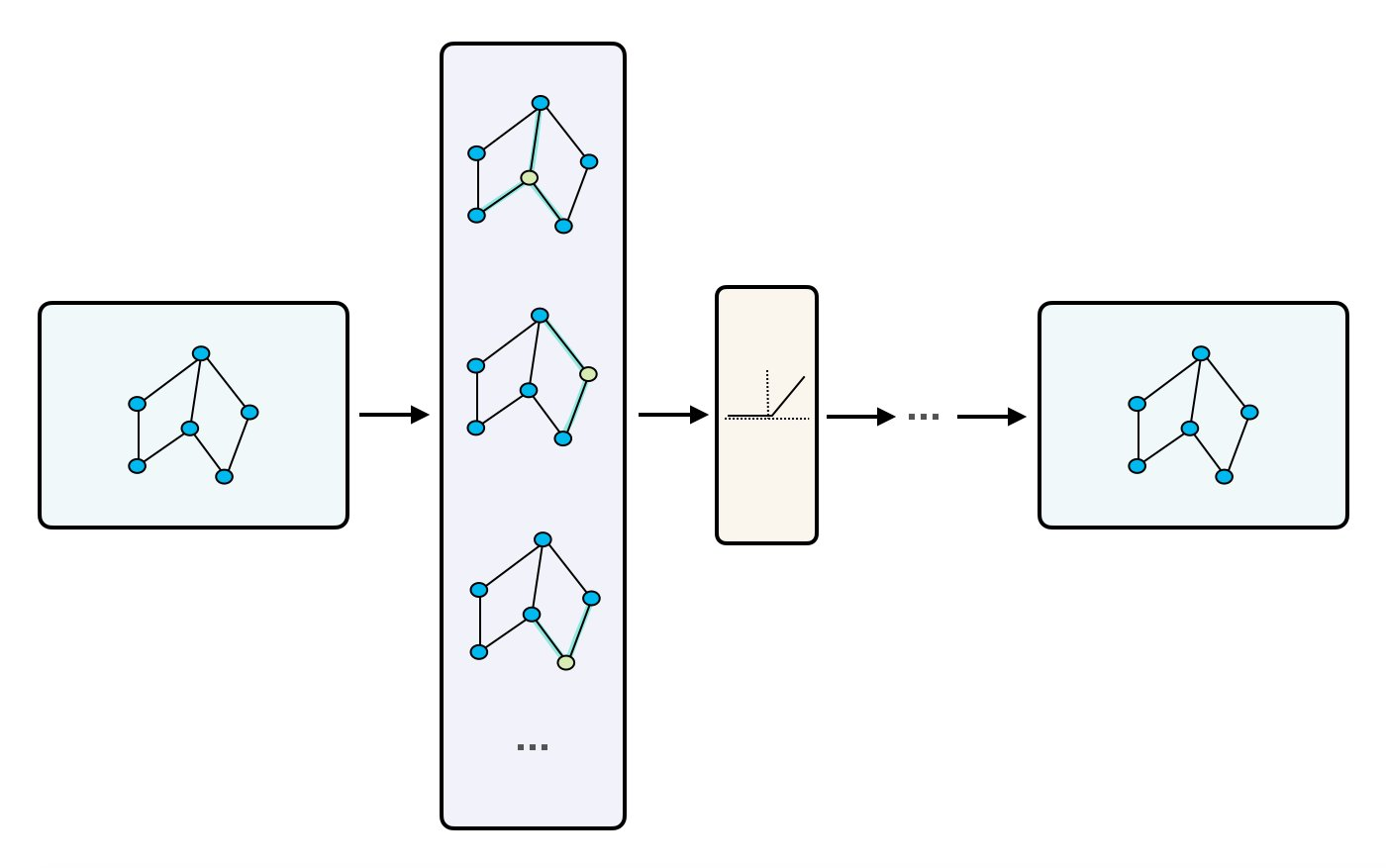## Overview

• Graph Neural Networks (GNNs) are a type of neural network architecture designed for processing graph-structured data. Graphs are a versatile data structure that can represent complex relationships, and GNNs extend traditional neural networks to operate directly on graphs.
• GNNs can aggregate information from neighboring nodes, capture local and global graph structures, and make predictions based on graph-structured data.
• They have been successfully applied to various applications, such as node classification, link prediction, and recommendation systems.
• Popular GNN architectures include Graph Convolutional Networks (GCNs), Graph Attention Networks (GATs), GraphSAGE, and GGNNs.
• GNNs are used in several industry applications such as maps for Google/Uber and social networks for LinkedIn, Instagram, and Facebook.
• GNNs are able to take advantage of both content information for entities (semantic), such as user and item features, as well as the underlying relationship between entities (structural), which may include user-item interactions or relationships with other entities, like sports. In contrast, traditional models often rely on just one of these sources of information, usually semantic.
• Implementing GNNs has been made easy by libraries such as PyTorch Geometric or Deep Graph Library.
• The image below (source) shows the different types of graphs. Note that recommender systems usually follow a bipartite graph.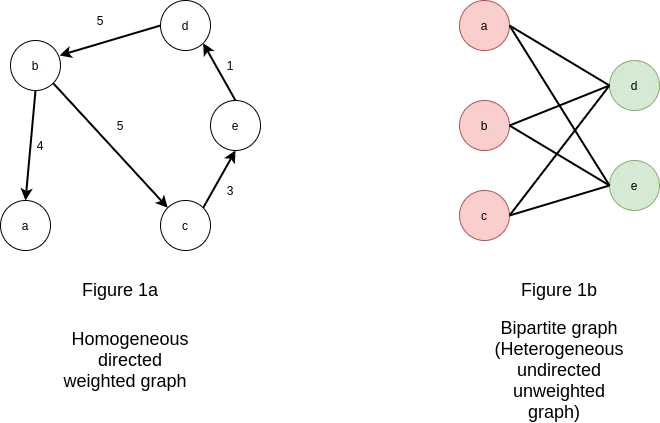## Graph Neural Networks

• Remember a graph is made up of sets of nodes or vertices that are connected by edges or links. At a high level, GNNs can be though of as an encoder-decoder architecture where the user and items are the nodes in the graph encoder and the edges represent the relationships or interactions between them.
• In the encoder phase, the graph is fed into the GNN, which computes a representation or embedding for each node based on its local neighborhood and the overall graph structure. This embedding can capture both the content or features of the node as well as its context within the graph.
• In the decoder phase, the GNN is used to make predictions or recommendations based on the learned node embeddings. This can involve computing similarity or distance measures between pairs of nodes, or using the embeddings as inputs to a downstream classifier or regression model.
• One advantage of using GNNs for recommender systems is that they can incorporate both explicit and implicit feedback from users, as well as contextual information such as time or location. Additionally, GNNs can be used to model complex relationships between users and items, such as multimodal interactions or hierarchical structures.
• The image below (source) shows a high level overview of how GNNs work for recommendation.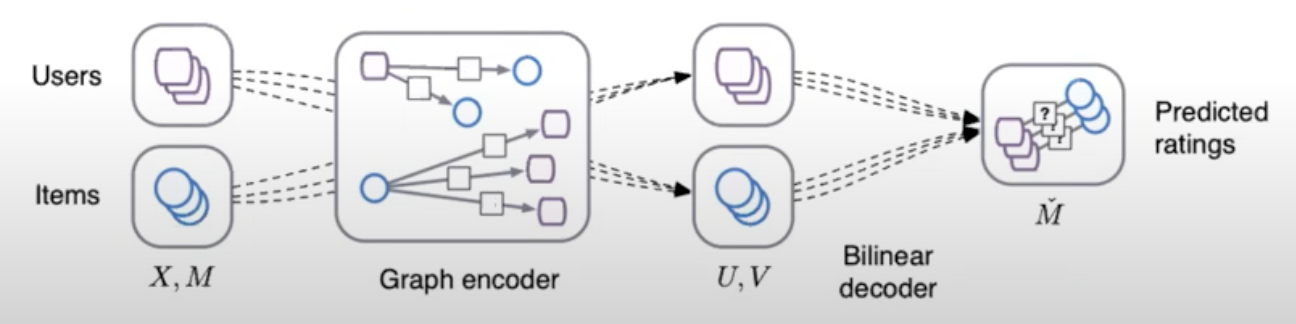• In GNN-based recommender systems, generating embeddings refers to the process of representing the user and item nodes in the graph as low-dimensional feature vectors that capture the relevant information about the node and its connections to other nodes in the graph. This is generally achieved through neural message passing.
• Finally, the prediction of preferences is done via using cosine similarity.

### Key terms

• Homogeneous Graphs: This graph type has all the nodes and edges be of the same type. An example is a social network, where all the users are nodes and the edges are their friendships.
• Heterogeneous Graphs: In contrast, this graph type has all the nodes and edges of different types with different representation of entities and relationships. An example is a recommendation system where users, movies, and genres are the entities, the graph would be considered heterogeneous because the nodes represent different types of objects (users, movies, genres) and the edges represent different types of relationships (user-movie interactions, movie-genre associations).
• Node Embeddings: Node embeddings are low-dimensional vector representations that capture the structural and relational information of nodes in a graph. GNNs are designed to learn these embeddings by iteratively aggregating information from neighboring nodes.
• Message Passing: Message passing is a fundamental operation in GNNs where nodes exchange information with their neighbors. During message passing, each node aggregates the information from its neighbors to update its own representation.
• Aggregation Functions: Aggregation functions are used in GNNs to combine information from neighboring nodes. Common aggregation functions include summation, averaging, and max-pooling, among others. These functions determine how information is aggregated and propagated through the graph.
• Graph Convolutional Networks (GCNs): GCNs are a popular type of GNN architecture that perform convolutional operations on graph-structured data. They adapt the concept of convolutions from traditional neural networks to the graph domain, enabling information propagation and feature extraction across the graph.
• GraphSAGE: GraphSAGE (Graph Sample and Aggregation) is a GNN model that uses sampling techniques to efficiently learn node embeddings. It aggregates information from sampled neighborhood nodes to update a node’s representation. GraphSAGE is commonly used in large-scale graph applications.
• Graph Attention Networks (GATs): GATs are GNN models that incorporate attention mechanisms. They assign attention weights to the neighboring nodes during message passing, allowing the model to focus on more relevant nodes and relationships.
• Link Prediction: Link prediction is a task in GNNs that aims to predict the presence or absence of edges between nodes in a graph. GNNs can learn to model the likelihood of missing or future connections based on the graph’s structure and node features.
• Graph Pooling: Graph pooling refers to the process of aggregating or downsampling nodes and edges in a graph to create a coarser representation. Pooling is often used in GNNs to handle graphs of varying sizes and reduce computational complexity.
• Graph Classification: Graph classification involves assigning a label or category to an entire graph. GNNs can be trained to perform graph-level predictions by aggregating information from the nodes and edges in the graph.
• Semi-Supervised Learning: GNNs often operate in a semi-supervised learning setting, where only a subset of nodes have labeled data. GNNs can leverage both labeled and unlabeled data to propagate information and make predictions on unlabeled nodes or graphs.

## GraphSAGE

• GraphSAGE (Graph Sample and Aggregated) is a GNN model introduced by Hamilton et al. in Inductive Representation Learning on Large Graphs. It aims to address the challenge of incorporating information from the entire neighborhood of a node in a scalable manner. The key idea behind GraphSAGE is to sample and aggregate features from a node’s local neighborhood to generate node representations.
• In GraphSAGE, each node aggregates feature information from its immediate neighbors. This aggregation is performed in a message-passing manner, where each node gathers features from its neighbors, performs a pooling operation (e.g., mean or max pooling) to aggregate the features, and then updates its own representation using the aggregated information. This process is repeated iteratively for multiple layers, allowing nodes to incorporate information from increasing distances in the graph.
• GraphSAGE utilizes the sampled and aggregated representations to learn node embeddings that capture both local and global graph information. These embeddings can then be used for various downstream tasks such as node classification or link prediction.

## Edge GNN

• Originally proposed in Exploiting Edge Features for Graph Neural Networks by Gong and Cheng from the University of Kentucky in CVPR 2019, Enhanced Graph Neural Network (EGNN) (or Edge GNN or Edge GraphSAGE) refers to an extension of the GraphSAGE model that incorporates information from both nodes and edges in the graph. While the original GraphSAGE focuses on aggregating information from neighboring nodes, Edge GNN takes into account the structural relationships between nodes provided by the edges.
• In Edge GNN, the message-passing process considers both the features of neighboring nodes and the features of connecting edges. This allows the model to capture more fine-grained information about the relationships between nodes, such as the type or strength of the edge connection. By incorporating edge features in addition to node features, Edge GNN can learn more expressive representations that capture both node-level and edge-level information.
• The Edge GNN model follows a similar iterative message-passing framework as GraphSAGE, where nodes gather and aggregate information from neighboring nodes and edges, update their representations, and propagate information across the graph. This enables the model to capture both semantic and structural information from both the local and global neighborhood of the graph and learn more comprehensive representations for nodes and edges.

### Embedding Generation: Neural Message Passing

• Generating embeddings in GNN is typically achieved through information propagation, also known as neural message passing, which involves passing information between neighboring nodes in the graph in a recursive manner, and updating the node representations based on the aggregated information.
• The propagation process allows the embeddings to capture both local and global information about the nodes, and to incorporate the contextual information from their neighbors.
• By generating informative and expressive embeddings, GNN-based recommenders can effectively capture the complex user-item interactions and item-item relations, and make accurate and relevant recommendations.
• Neural message passing is a key technique for generating embeddings in GNN-based recommender systems. It allows the nodes in the graph to communicate with each other by passing messages along the edges, and updates their embeddings based on the aggregated information.
• At a high level, the message passing process consists of two steps:
1. Message computation: In this step, each node sends a message to its neighboring nodes, which is typically computed as a function of the node’s own embedding and the embeddings of its neighbors. The message function can be a simple linear transformation, or a more complex non-linear function such as a neural network.
2. Message aggregation: In this step, each node collects the messages from its neighbors and aggregates them to obtain a new representation of itself. The aggregation function can also be a simple sum or mean, or a more complex function such as a max-pooling or attention mechanism.
• The message passing process is usually performed recursively for a fixed number of iterations, allowing the nodes to exchange information with their neighbors and update their embeddings accordingly. The resulting embeddings capture the local and global information about the nodes, as well as the contextual information from their neighbors, which is useful for making accurate and relevant recommendations.
• Some common algorithms and techniques used for neural message passing in GNNs are:
• Graph Convolutional Networks (GCNs): GCNs apply a localized convolution operation to each node in the graph, taking into account the features of its neighboring nodes. This allows for the aggregation of information from neighboring nodes to update the node’s feature representation.
• Graph Attention Networks (GATs): GATs use a learnable attention mechanism to weigh the importance of neighboring nodes when updating a node’s feature representation. This allows the model to selectively focus on the most relevant neighbors.
• GraphSAGE: GraphSAGE uses a hierarchical sampling scheme to aggregate information from the neighborhood of each node. This allows for efficient computation of node embeddings for large graphs.
• Message Passing Neural Networks (MPNNs): MPNNs use a general framework for message passing between nodes in a graph, allowing for flexibility in modeling different types of interactions.
• In the context of GNNs for recommender systems, the goal is to generate embeddings for the user and item nodes in the graph. The embeddings can then be used for tasks such as candidate generation, scoring, and ranking.
• The process of generating embeddings involves multiple GNN layers, each of which performs an exchange of information between the immediate neighbors in the graph. At each layer, the information exchanged is aggregated and processed to generate new embeddings for each node. This process can be repeated for as many layers as desired, and the number of layers determines how far information is propagated in the graph.
• For example, in a 2-layer GNN model, each node will receive information from its immediate neighbors (i.e., nodes connected by an edge) and its immediate neighbors’ neighbors. This allows information to be propagated beyond a node’s direct neighbors, potentially capturing higher-level structural relationships in the graph.
• From Building a Recommender System Using Graph Neural Networks, here is the pseudo-code for generating embeddings for a given node:
1. Fetch incoming messages from all neighbors.
2. Reduce all those messages into 1 message by doing mean aggregation.
3. Matrix multiplication of the neighborhood message with a learnable weight matrix.
4. Matrix multiplication of the initial node message with a learnable weight matrix.
5. Sum up the results from steps 3 and 4.
6. Pass the sum through a ReLU activation function to model non-linearity relationships in the data.
7. Repeat for as many layers as desired. The result is the output of the last layer.
• The image below (source), visually represents this pseudo code.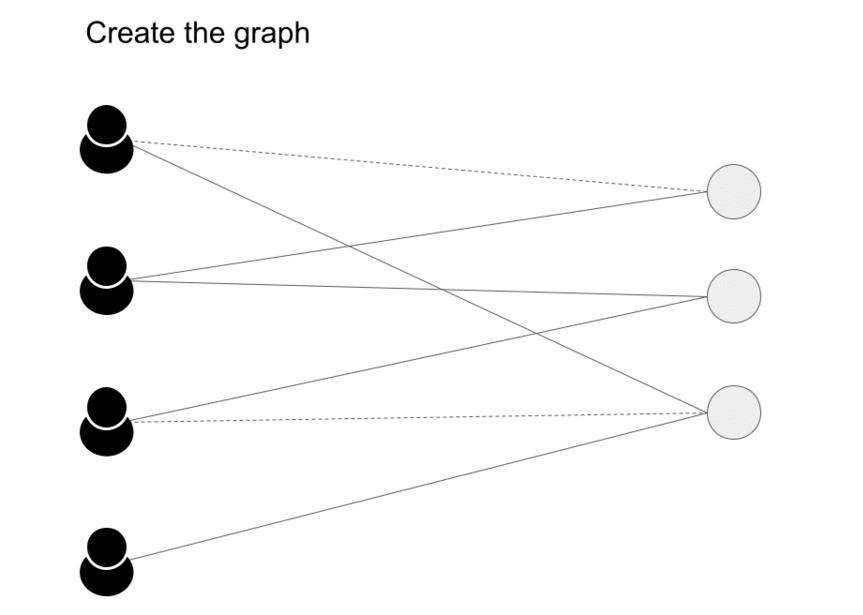• Message passing has two steps, Aggregation and Update as we can see in the image (source) below.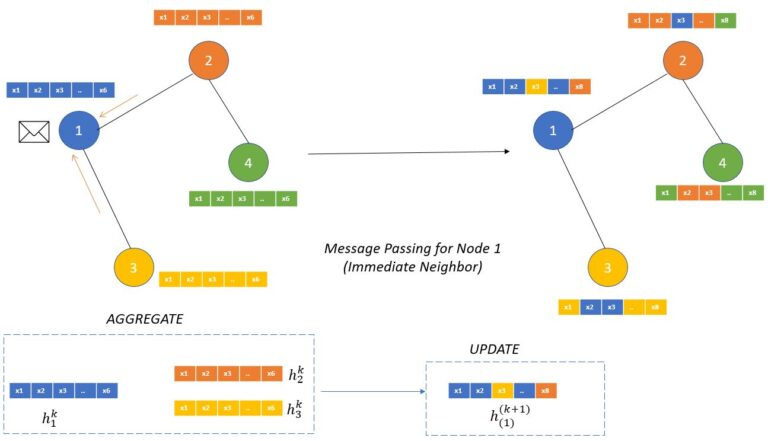• The aggregation function works on defining how the messages from the neighboring nodes are combined to compute new representations of the node.
• “This aggregate function should be a permutation invariant function like sum or average. The update function itself can be a neural network (with attention or without attention mechanism) which will generate the updated node embeddings.” (source)

## Benefits of GNN

• Incorporating Graph Structure: GNNs are designed to process data with inherent graph structure, which is particularly useful in recommender systems. Recommender systems often involve modeling relationships between users, items, and their interactions. GNNs can effectively capture these complex relationships and dependencies by leveraging the graph structure, leading to more accurate and personalized recommendations.
• Implicit Collaborative Filtering: Collaborative filtering is a popular recommendation technique that relies on user-item interactions. GNNs can handle implicit feedback data, such as user clicks, views, or purchase history, without the need for explicit ratings. GNNs can learn from the graph connections and propagate information across users and items, enabling collaborative filtering in a more efficient and scalable manner.
• Modeling User and Item Features: GNNs can handle heterogeneous data by incorporating user and item features alongside the graph structure. In recommender systems, users and items often have associated attributes or contextual information that can influence the recommendations. GNNs can effectively integrate these features into the learning process, allowing for more personalized recommendations that consider both user preferences and item characteristics.
• Capturing Higher-Order Dependencies: GNNs can capture higher-order dependencies by aggregating information from neighboring nodes in multiple hops. This allows GNNs to capture complex patterns and relationships that may not be easily captured by traditional recommendation algorithms. GNNs can discover latent factors and capture long-range dependencies, resulting in improved recommendation quality.
• Cold Start Problem: GNNs can help address the cold start problem, which occurs when there is limited or no historical data for new users or items. By leveraging the graph structure and user/item features, GNNs can generalize from existing data and make reasonable recommendations even for users or items with limited interactions.
• Interpretability: GNNs provide interpretability by allowing inspection of the learned representations and the influence of different nodes or edges in the graph. This can help understand the reasoning behind recommendations and provide transparency to users, increasing their trust in the system.

## Loss functions

• Binary Cross-Entropy Loss: Binary cross-entropy loss is often used for binary classification tasks in GNNs. It is suitable when the task involves predicting a binary label or making a binary decision based on the graph structure and node features.
• Categorical Cross-Entropy Loss: Categorical cross-entropy loss is used for multi-class classification tasks in GNNs. If the GNN is trained to predict the class label of nodes or edges in a graph, this loss function is commonly employed.
• Mean Squared Error (MSE) Loss: MSE loss is frequently used for regression tasks in GNNs. If the goal is to predict a continuous or numerical value associated with nodes or edges in the graph, MSE loss can measure the difference between predicted and true values.
• Pairwise Ranking Loss: Pairwise ranking loss is suitable for recommendation or ranking tasks in GNNs. It is used when the goal is to learn to rank items or nodes based on their relevance or preference to users. Examples of pairwise ranking loss functions include the hinge loss and the pairwise logistic loss.
• Triplet Ranking Loss: Triplet ranking loss is another type of loss function used for ranking tasks in GNNs. It aims to learn representations that satisfy certain constraints among a triplet of samples. The loss encourages the model to assign higher rankings to relevant items compared to irrelevant items.
• Graph Reconstruction Loss: Graph reconstruction loss is employed when the goal is to reconstruct the input graph or its properties using the GNN. This loss compares the reconstructed graph with the original graph to measure the similarity or reconstruction error.

## Graph Neural Networks (GNNs) in NLP

• Graph Neural Networks (GNNs) are a type of Neural Network that operate on data structured as graphs. They can capture the complex relationships between nodes in a graph, which can often provide more nuanced representations of the data than traditional neural networks.
• While GNNs are often used in areas like social network analysis, citation networks, and molecular chemistry, they’re also finding increasing use in the field of Natural Language Processing (NLP).
• One of the main ways GNNs are used in NLP is in capturing the dependencies and relations between words in a sentence or phrases in a text. For example, a text can be represented as a graph where each word is a node and the dependencies between them are edges. GNNs can then learn representations for the words in a way that takes into account both the words’ individual properties and their relations to other words.
• One specific use case is in the field of Information Extraction, where GNNs can help determine relations between entities in a text. They can also be used in semantic role labeling, where the aim is to understand the semantic relationships between the words in a sentence.
• The fundamental principle behind GNNs is the neighborhood aggregation or message-passing framework. The representation of a node in a graph is computed by aggregating the features of its neighboring nodes. The most basic form of GNN - Graph Convolutional Network (GCN) operates in the following way:
1. Node-level Feature Aggregation:
• For each node $$v$$ in the graph, we aggregate the feature vectors of its neighboring nodes. This can be a simple average: $$h_{v}^{(1)} = \frac{1}{|\mathcal{N}(v)|} \sum_{u \in \mathcal{N}(v)} x_u$$
• where $$h_{v}^{(1)}$$ is the first-level feature of node $$v$$, $$x_u$$ is the input feature of node $$u$$, and $$\|\mathcal{N}(v)\|$$ denotes the number of neighbors of $$v$$.
2. Feature Transformation:
• Then, a linear transformation followed by a non-linear activation function is applied to these aggregated features: $$h_{v}^{(2)} = \sigma(W h_{v}^{(1)})$$
• where $$h_{v}^{(2)}$$ is the second-level feature of node $$v$$, $$W$$ is a learnable weight matrix, and $$\sigma$$ denotes a non-linear activation function such as ReLU.

## Loss Function for GNNs in NLP

• The choice of loss function in training GNNs for NLP tasks depends on the specific task at hand.
• For example, in a classification task (like sentiment analysis), you might use a cross-entropy loss, which measures the dissimilarity between the predicted probability distribution and the true distribution.
• In a sequence labeling task (like named entity recognition or part-of-speech tagging), you might use a sequence loss like the conditional random field (CRF) loss.
• In a regression task (like predicting the semantic similarity between two sentences), you might use a mean squared error loss.
• It’s also common to use a combination of different loss functions. For example, you might use a combination of cross-entropy loss for classification and a regularization term to prevent overfitting.
• In all cases, the goal of the loss function is to provide a measure of how well the network’s predictions align with the true labels in the training data, and to guide the adjustment of the network’s weights during training.
• The choice of loss function for GNNs in NLP tasks greatly depends on the specific problem being addressed.

• Classification Task: For a multi-class classification task, Cross-Entropy loss is commonly used. The cross-entropy loss for a single data point can be calculated as:

$L = -\sum_{c=1}^{M} y_{o,c} \log(p_{o,c})$
• where $$y$$ is a binary indicator (0 or 1) if class label $$c$$ is the correct classification for observation $$o$$, and $$p$$ is the predicted probability observation $$o$$ is of class $$c$$.
• Sequence Labeling Task: For tasks like named entity recognition or part-of-speech tagging, Conditional Random Field (CRF) loss can be used. This loss is more complicated and beyond the scope of this brief explanation, but essentially it learns to predict sequences of labels that take into account not just individual label scores, but also their relationships to each other.

• Regression Task: For predicting continuous values, Mean Squared Error (MSE) loss is commonly used. The MSE loss can be calculated as:

$L = \frac{1}{n}\sum_{i=1}^{n}(Y_i - \hat{Y_i})^2$
• where $$Y$$ is the ground truth value, $$Ŷ$$ is the predicted value, and $$n$$ is the total number of data points.
• It’s important to note that these are just typical examples. The choice of loss function should always be tailored to the specific task and the data at hand.

## Walkthrough

• Now, let’s do a quick walkthrough on creating our own system from scratch and see all the steps that it would take.
• First is the dataset, say we have user-item interaction, item features and user features available as shown below (source) starting with user-item interaction.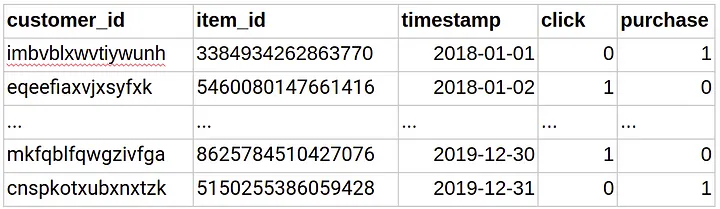• The item features are as below.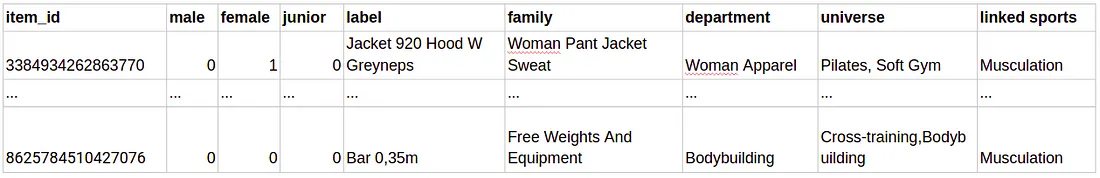• The user features are as below.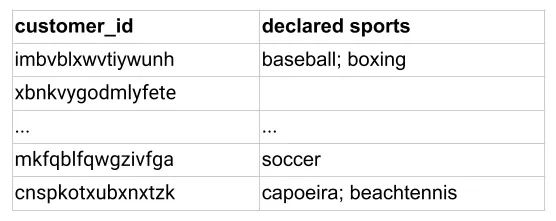• The next step is to create a graph as shown below (source).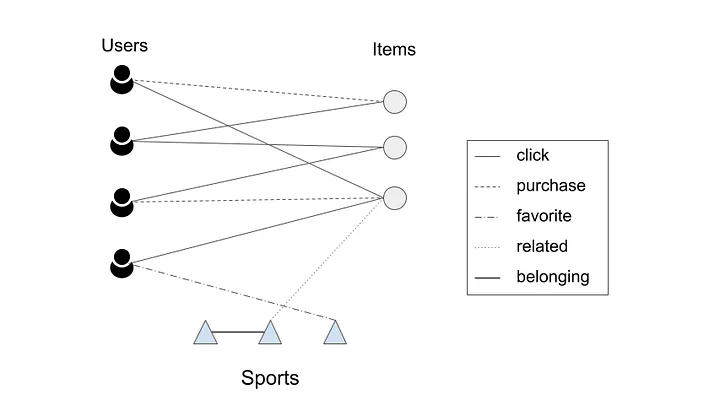• The embeddings are created using the procedure elaborated in Embedding Generation: Neural Message Passing. The embeddings generated by GNNs are utilized to estimate the likelihood of a connection between two nodes. To calculate the probability of interaction between a user $$u$$ and an item $$v$$, we use the cosine similarity function. After computing scores for all items that a user did not interact with, the system recommends the items with the highest scores.
• The main goal during training of the model is to optimize the trainable matrices ($$W$$) used for generating the embeddings. To achieve this, a max-margin loss function is used, which involves negative sampling. The training data set only includes edges representing click and purchase events.
• The model is trained in such a way that it learns to predict a higher score for a positive edge (an edge between a user and an item that they actually interacted with) compared to randomly sampled negative edges. These negative edges are connections between a user and random items that they did not interact with. The idea is to teach the model to distinguish between actual positive interactions and randomly generated negative interactions.

### GNN Papers and Implementations

• If you want to keep up-to-date with popular recent methods and paper implementations for GNNs, the Papers with Code community maintains this useful collection:

### Benchmarks and Datasets

• If you are interested in benchmarks/leaderboards and graph datasets that evaluate GNNs, the Papers with Code community also maintains such content here:

## Tools

• Here are a few useful tools to get started with GNNs:

## Citation

If you found our work useful, please cite it as:

@article{Chadha2020DistilledGraphNeuralNetworks,
title   = {Graph Neural Networks},